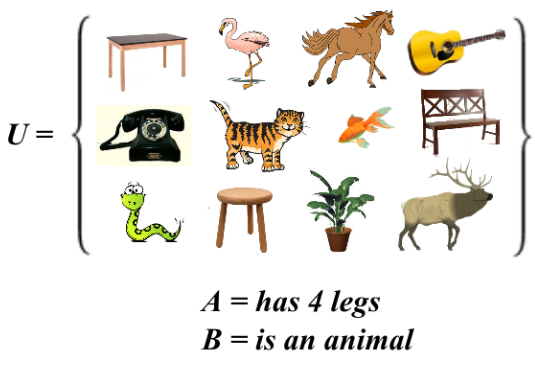# Legs?

Probability Level 2What is $|A^{C}\cup B^{C}|$?

Details:

$|S|$ represents the cardinality of the set $S$ and $S^C$ represents the complement of the set $S$.

×